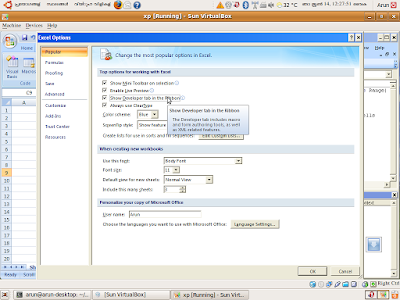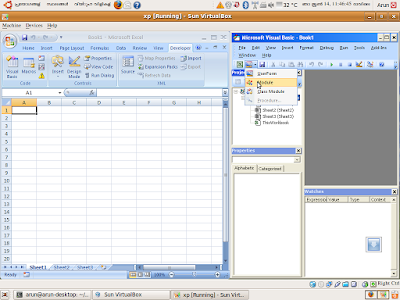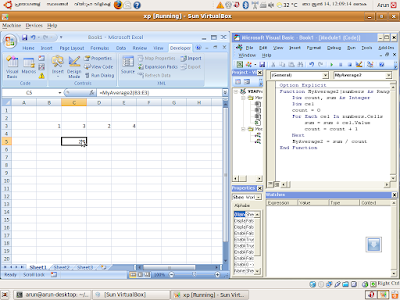# Writing custom formulaes for excel using VBA

It will be easy to if we are able to add our own formulaes in excel. The following steps explains how to add a formulae using VBA (Excel Macros).
First open the visual basic editor ( In excel 2003 it is available from Tools>Macros>visual basic editor. In excel 2007 it should be enabled  from the excel options menu, then invoke using Alt+F11 or from the developer ribbon)Enabling of Visual Basic Editor in Exel 2007In the visual basic editor, add a new moduleType the function as shown in the screen shot.
This function will be vailable as a formulae in excel sheet.
In the example shown above, the formulae MyAverage1 is defined which will calculate the average of three numbers.In this example the MyAverage2 is made which will calculate the average for a range of cells.

If it is necessary to create a library of formulaes, then save the exec as an excel addin. The put the addin in the "C:\Documents and Settings\\Application Data\Microsoft\AddIns" folder. Now select the addin from Tools>addins menu for execl 2003 or Exec options>addins menu for excel 2003